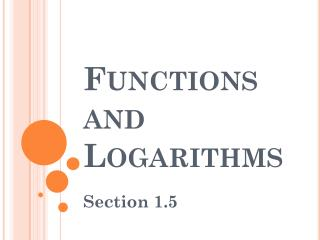DownloadDownload PresentationFunctions and Logarithms

# Functions and Logarithms

Download Presentation## Functions and Logarithms

- - - - - - - - - - - - - - - - - - - - - - - - - - - E N D - - - - - - - - - - - - - - - - - - - - - - - - - - -
##### Presentation Transcript

1. Functions and Logarithms Section 1.5

2. First, some basic review… What does the Vertical Line Test tell us? Whether or not the graph of a relation is a function… What does the Horizontal Line Test tell us? Whether or not a relation’s inverse is a function… A function that passes both the VLT and HLT is called: One-to-One

3. One-to-One Functions

4. Inverses • Since each output of a one-to-one function comes from just one input, a one-to-one function can be reversed to send outputs back to the inputs from which they came. • The function defined by reversing a one-to-one function f is the inverse of f. • Composing a function with its inverse in either order sends each output back to the input from which it came.

5. Inverses

6. Inverses Find and verify that First, solve for x: Interchange x and y: Verification: (in the given domains)

7. Logarithmic Functions Switching between exponential and logarithmic form: The key element of this “linking” statement: Logarithms ARE Exponents!!!

8. Logarithmic Functions • Logarithms with base e and base 10 are so important in applications that calculators have special keys for them. • They also have their own special notations and names.

9. Logarithmic Functions How to “read” logarithmic expressions… “b to what power is x?” “Read” each properly, then evaluate: “2 to what power is 8?” “10 to what power is 1/100?” 5 “e to what power is e ?”

10. Properties of Logarithms Change of Base (COB) Formula

11. Guided Practice Find a formula for Switch x and y, solve for y:

12. Guided Practice Solve the equation algebraically. Support graphically. Does the graph support our analytic answer?

13. Guided Practice Solve the equation algebraically. Support graphically. This equation is quadratic in ...

14. Guided Practice Solve the equation algebraically. Support graphically. Do both of these answers work? Graphical support?